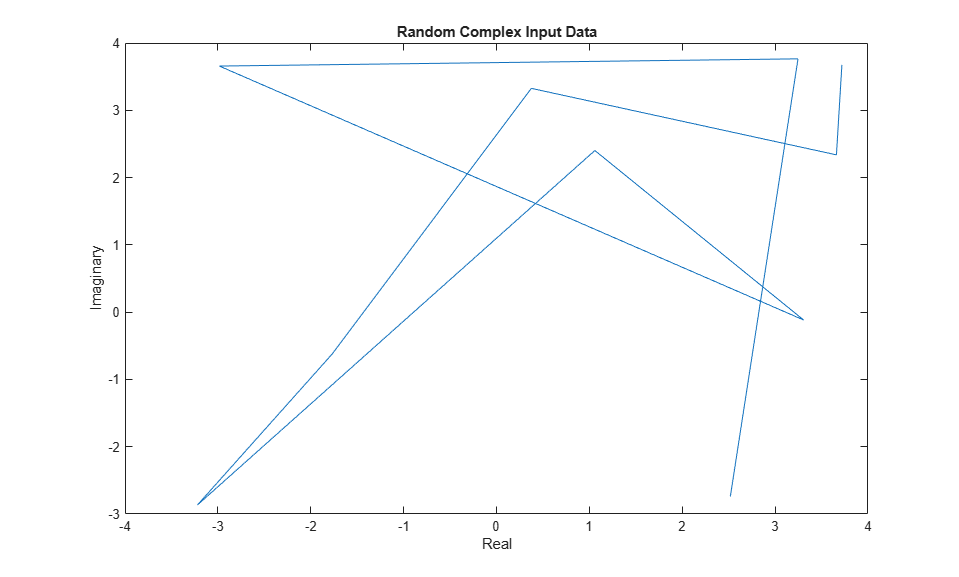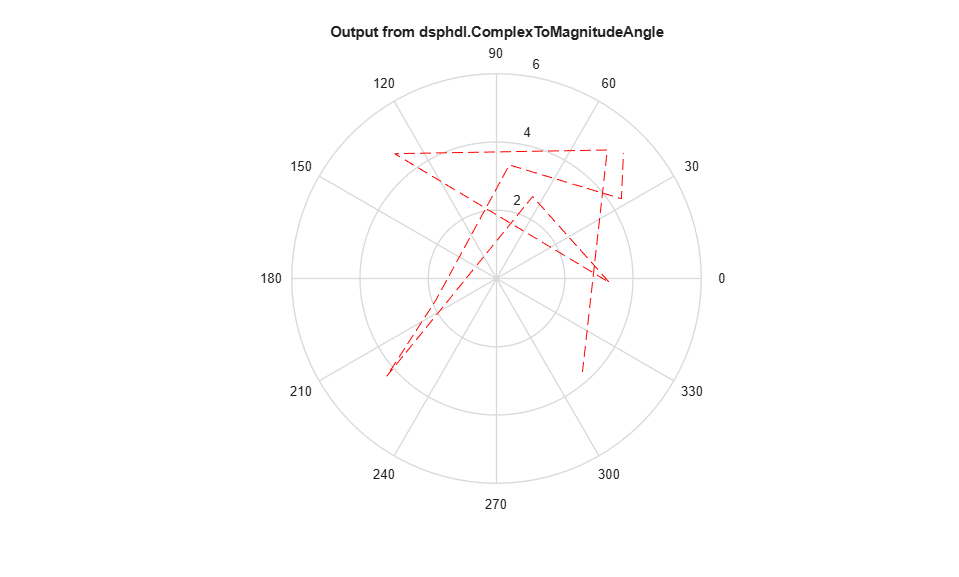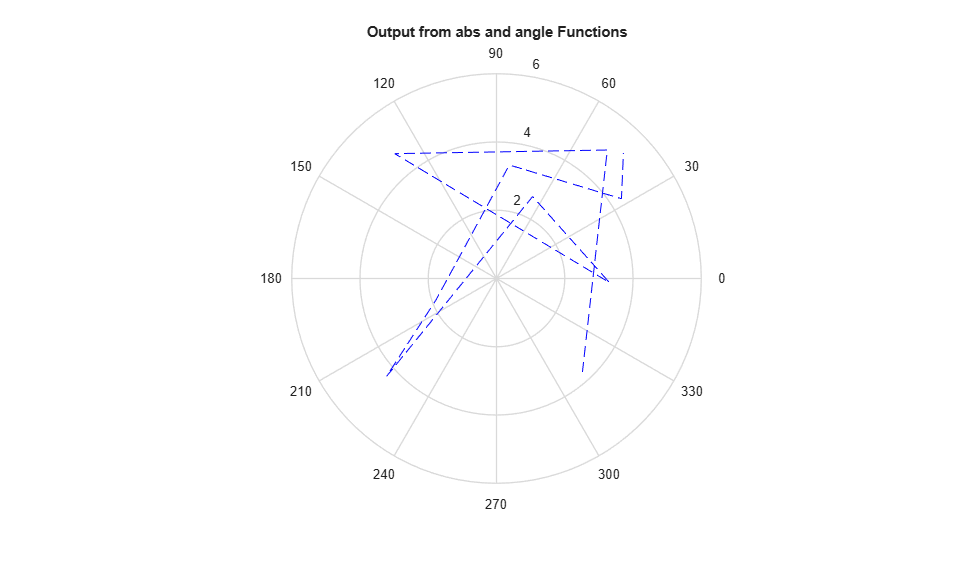# dsphdl.ComplexToMagnitudeAngle

Magnitude and phase angle of complex signal

## Description

The `dsphdl.ComplexToMagnitudeAngle` System object™ computes the magnitude and phase angle of a complex signal. It provides hardware-friendly control signals. The System object uses a pipelined coordinate rotation digital computer (CORDIC) algorithm to achieve an HDL-optimized implementation.

To compute the magnitude and phase angle of a complex signal:

1. Create the `dsphdl.ComplexToMagnitudeAngle` object and set its properties.

2. Call the object with arguments, as if it were a function.

## Creation

### Syntax

``magAngle = dsphdl.ComplexToMagnitudeAngle``
``magAngle = dsphdl.ComplexToMagnitudeAngle(Name=Value)``

### Description

````magAngle = dsphdl.ComplexToMagnitudeAngle` returns a `dsphdl.ComplexToMagnitudeAngle` System object, `magAngle`, that computes the magnitude and phase angle of a complex input signal.```

example

````magAngle = dsphdl.ComplexToMagnitudeAngle(Name=Value)` sets properties of the `magAngle` object using one or more name-value arguments. Example: ```magAngle = dsphdl.ComplexToMagnitudeAngle(AngleFormat='Radians')``````

## Properties

expand all

Unless otherwise indicated, properties are nontunable, which means you cannot change their values after calling the object. Objects lock when you call them, and the `release` function unlocks them.

If a property is tunable, you can change its value at any time.

Type of output values to return, specified as ```'Magnitude and angle'```, `'Magnitude'`, or `'Angle'`. You can choose for the object to return the magnitude of the input signal, or the phase angle of the input signal, or both.

Format of the phase angle output value from the object, specified as:

• `'Normalized'` — Fixed-point format that normalizes the angle in the range [–1,1].

• `'Radians'` — Fixed-point values in the range [π,−π].

Scale output by the inverse of the CORDIC gain factor, specified as `true` or `false`. The object implements this gain factor with either CSD logic or a multiplier, according to the `ScalingMethod` property.

Note

If your design includes a gain factor later in the datapath, you can set `ScaleOutput` to `false`, and include the CORDIC gain factor in the later gain. For calculation of this gain factor, see Algorithm. The object replaces the first CORDIC iteration by mapping the input value onto the angle range [0,π/4]. Therefore, the initial rotation does not contribute a gain term.

Source of the `NumIterations` property for the CORDIC algorithm, specified as:

• `'Auto'` — Sets the number of iterations to one less than the input word length. If the input is `double` or `single`, the number of iterations is 16.

• `'Property'` — Uses the `NumIterations` property.

For details of the CORDIC algorithm, see Algorithm.

Number of CORDIC iterations that the object executes, specified as an integer. The number of iterations must be less than or equal to one less than the input word length.

For details of the CORDIC algorithm, see Algorithm.

#### Dependencies

To enable this property, set `NumIterationsSource` to `'Property'`.

When you set this property to `'CSD'`, the object implements the CORDIC gain scaling by using a shift-and-add architecture for the multiply operation. This implementation uses no multiplier resources and may increase the length of the critical path in your design. When you set this property to `'Multipliers'`, the object implements the CORDIC gain scaling with a multiplier and increases the latency of the object by four cycles.

#### Dependencies

To enable this property, set the `ScaleOutput` property to `true`.

## Usage

### Syntax

``````[mag,angle,validOut] = magAngle(X,validIn)``````
``````[mag,validOut] = magAngle(X,validIn)``````
``````[angle,validOut] = magAngle(X,validIn)``````

### Description

example

``````[mag,angle,validOut] = magAngle(X,validIn)``` converts a scalar or vector of complex values `X` into their component magnitude and phase angles. `validIn` and `validOut` are logical scalars that indicate the validity of the input and output signals, respectively.```
``````[mag,validOut] = magAngle(X,validIn)``` returns only the component magnitudes of `X`. To use this syntax, set `OutputFormat` to `'Magnitude'`. Example: ```magAngle = dsphdl.ComplextoMagnitudeAngle(OutputFormat='Magnitude');``````
``````[angle,validOut] = magAngle(X,validIn)``` returns only the component phase angles of `X`.To use this syntax, set `OutputFormat` to `'Angle'`. Example: ```magAngle = dsphdl.ComplextoMagnitudeAngle(OutputFormat='Angle');``````

### Input Arguments

expand all

Input signal, specified as a scalar, a column vector representing samples in time, or a row vector representing channels. Using vector input increases data throughput while using more hardware resources. The object implements the conversion logic in parallel for each element of the vector. The input vector can contain up to 64 elements.

The software supports `double` and `single` data types for simulation, but not for HDL code generation.

Data Types: `fi` | `int8` | `int16` | `int32` | `uint8` | `uint16` | `uint32` | `single` | `double`
Complex Number Support: Yes

Control signal that indicates if the input data is valid. When `validIn` is `1` (`true`), the object captures the values from the `dataIn` argument. When `validIn` is `0` (`false`), the object ignores the values from the `dataIn` argument.

Data Types: `logical`

### Output Arguments

expand all

Magnitude calculated from the complex input signal, returned as a scalar, a column vector representing samples in time, or a row vector representing channels. The dimensions and data type of this argument match the dimensions of the `dataIn` argument.

#### Dependencies

To enable this argument, set the `OutputFormat` property to `'Magnitude and Angle'` or `'Magnitude'`.

Angle calculated from the complex input signal, returned as a scalar, a column vector representing samples in time, or a row vector representing channels. The dimensions and data type of this argument match the dimensions of the `dataIn` argument. The format of this value depends on the `AngleFormat` property.

#### Dependencies

To enable this argument, set the `OutputFormat` property to `'Magnitude and Angle'` or `'Angle'`.

Control signal that indicates if the output data is valid. When `validOut` is `1` (`true`), the object returns valid data from the `mag` and/or `angle` arguments. When `validOut` is `0` (`false`), values from the `mag` and/or `angle` arguments are not valid.

Data Types: `logical`

## Object Functions

To use an object function, specify the System object as the first input argument. For example, to release system resources of a System object named `obj`, use this syntax:

`release(obj)`

expand all

 `step` Run System object algorithm `release` Release resources and allow changes to System object property values and input characteristics `reset` Reset internal states of System object

## Examples

collapse all

Use the `dsphdl.ComplextoMagnitudeAngle` object to compute the magnitude and phase angle of a complex signal. The object uses a CORDIC algorithm for an efficient hardware implementation.

Choose word lengths and create random complex input signal. Then, convert the input signal to fixed-point.

```a = -4; b = 4; inputWL = 16; inputFL = 12; numSamples = 10; reData = ((b-a).*rand(numSamples,1)+a); imData = ((b-a).*rand(numSamples,1)+a); dataIn = (fi(reData+imData*1i,1,inputWL,inputFL)); figure plot(dataIn) title('Random Complex Input Data') xlabel('Real') ylabel('Imaginary') ```Write a function that creates and calls the System object™. You can generate HDL from this function.

```function [mag,angle,validOut] = Complex2MagAngle(yIn,validIn) %Complex2MagAngle % Converts one sample of complex data to magnitude and angle data. % yIn is a fixed-point complex number. % validIn is a logical scalar value. % You can generate HDL code from this function. persistent cma; if isempty(cma) cma = dsphdl.ComplexToMagnitudeAngle(AngleFormat='Radians'); end [mag,angle,validOut] = cma(yIn,validIn); end % Copyright 2021-2023 The MathWorks, Inc. ```

The number of CORDIC iterations determines the latency that the object takes to compute the answer for each input sample. The latency is `NumIterations+4`. In this example, `NumIterationsSource` is set to the default, `'Auto'`, so the object uses `inputWL-1` iterations. The latency is `inputWL+3`.

```latency = inputWL+3; mag = zeros(1,numSamples+latency); ang = zeros(1,numSamples+latency); validOut = false(1,numSamples+latency); ```

Call the function to convert each sample. After you apply all input samples, continue calling the function with invalid input to flush remaining output samples.

```for ii = 1:1:numSamples [mag(ii),ang(ii),validOut] = Complex2MagAngle(dataIn(ii),true); end for ii = (numSamples+1):1:(numSamples+latency) [mag(ii),ang(ii),validOut(ii)] = Complex2MagAngle(fi(0+0*1i,1,inputWL,inputFL),false); end % Remove invalid output values mag = mag(validOut == 1); ang = ang(validOut == 1); figure polar(ang,mag,'--r') % Red is output from System object title('Output from dsphdl.ComplexToMagnitudeAngle') magD = abs(dataIn); angD = angle(dataIn); figure polar(angD,magD,'--b') % Blue is output from abs and angle functions title('Output from abs and angle Functions') ```expand all

## Version History

Introduced in R2014b

expand all